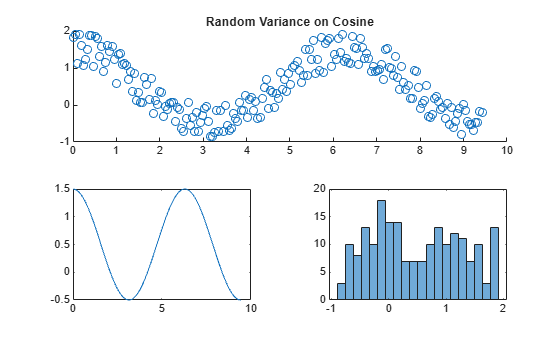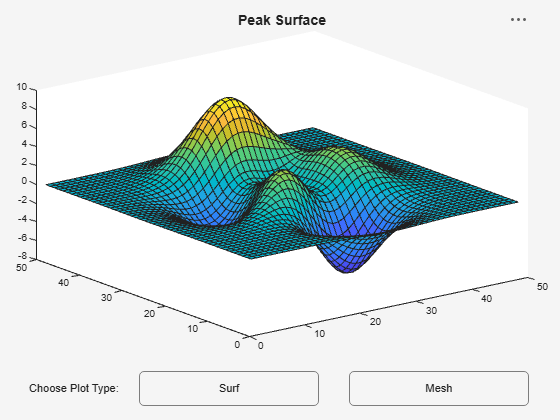# fontsize

Change font size for objects in a figure

## Syntax

``fontsize(obj,size,units)``
``fontsize(obj,"increase")``
``fontsize(obj,"decrease")``
``fontsize(obj,scale=sfactor)``
``fontsize(obj,"default")``

## Description

example

````fontsize(obj,size,units)` sets the font size and font units for all of the text within graphics object `obj`. If `obj` contains other graphics objects, such as a figure that contains UI components or an axes object that has a legend, `fontsize` also sets the font size and font units for those objects within `obj`. Font units can be `"points"`, `"pixels"`, `"inches"`, or `"centimeters"`.```

example

````fontsize(obj,"increase")` increases the font size by a factor of 1.1.```
````fontsize(obj,"decrease")` decreases the font size by a factor of 0.9.```

example

````fontsize(obj,scale=sfactor)` scales the font size by a factor of `sfactor`. For example, use a scale factor of `1.2` to scale by 120%.```

example

````fontsize(obj,"default")` resets the font size and font units back to the default, automatic values for `obj`.```

## Examples

collapse all

Create a plot of random data, and add a plot title and legend.

```y = rand(5); plot(y) title("2-D Line Plot") legend("Line 1","Line 2","Line 3","Line 4","Line 5")```Set the font size of all text within the axes object to `10` and the font units to `"pixels"`. Use the object returned by `gca` as the target object to apply this change.

`fontsize(gca,10,"pixels")`Create a plot with several text elements of varying font sizes.

```[X,Y,Z] = peaks; contourf(X,Y,Z,LineColor="#4F4F4F") title("Peak Elevation") colorbar annotation("textarrow",[.53 .41],[.65 .47],String="Local maxima") annotation("textarrow",[.53 .59],[.65 .55])```Scale the font sizes in the figure by 120% by using a scale factor of 1.2. The `fontsize` function scales each font size individually, maintaining the relative sizes of the fonts.

`fontsize(gcf,scale=1.2)`Create a tiling of several plots by using the `tiledlayout` and `nexttile` functions.

```x = linspace(0,3*pi,200); y = cos(x) + rand(1,200); t = tiledlayout(2,2); % Top scatter plot in tiles 1,2 ax1 = nexttile([1 2]); scatter(x,y) title("Random Variance on Cosine") % Lower polar plot in tile 3 ax2 = nexttile; plot(x,cos(x)+0.5) % Lower histogram in tile 4 ax3 = nexttile; histogram(y,20)```Scale up the font size of the scatter plot, and change the font size of the other two plots to 10 pixels.

```fontsize(ax1,scale=1.2) fontsize([ax2 ax3],10,"pixels")```To undo the font size changes across all the tiled plots, reset the font sizes and units to their default values. Apply this change to all three plots by using the current figure object returned by `gcf`.

`fontsize(gcf,"default")`Create the following function file, and save it as `myapplayout.m` on your MATLAB® path. This function returns the layout for a simple app to plot data using different plot types.

```function fig = myapplayout % Create figure window fig = uifigure; % Create UI components ax = uiaxes(fig,Position=[15 70 535 340]); lbl = uilabel(fig,Position=[30 15 100 35],Text="Choose Plot Type:"); b1 = uibutton(fig,Position=[140 15 180 35],Text="Surf"); b2 = uibutton(fig,Position=[350 15 180 35],Text="Mesh"); % Configure UI component appearance surf(ax,peaks); fontsize(fig,8,"pixels") title(ax,"Peak Surface",FontSize=11) end ```

Call the function and assign the returned figure object to `f`.

`f = myapplayout;`Use `f` to increase the font size of all text in the figure until it is more easily readable. Here, the `fontsize` function increases each font size individually by a scale factor of 1.1, maintaining the relative sizes of the fonts.

```fontsize(f,"increase") fontsize(f,"increase") fontsize(f,"increase")```## Input Arguments

collapse all

Object or container with text, specified as a graphics object or array of graphics objects. The `fontsize` function sets the font size of text in the specified objects. If `obj` contains other graphics objects, such as a figure that contains UI components or an axes object that has a legend, the function also sets the font size and font units for those objects within `obj`. Objects without a `FontSize` property are not affected.

Font size, specified as a positive scalar value.

Data Types: `single` | `double` | `int8` | `int16` | `int32` | `int64` | `uint8` | `uint16` | `uint32` | `uint64`

Font size units, specified as `"points"`, `"pixels"`, `"inches"`, or `"centimeters"`.

Scale factor, specified as a scalar. All font sizes under the target object are scaled by `sfactor`. Use a scale factor greater than `1` to increase the font sizes or a factor less than `1` to decrease the font sizes.

Example: `fontsize(gcf,scale=0.8)` scales the text to 80% of its original size.

Data Types: `single` | `double` | `int8` | `int16` | `int32` | `int64` | `uint8` | `uint16` | `uint32` | `uint64`

## Version History

Introduced in R2022a